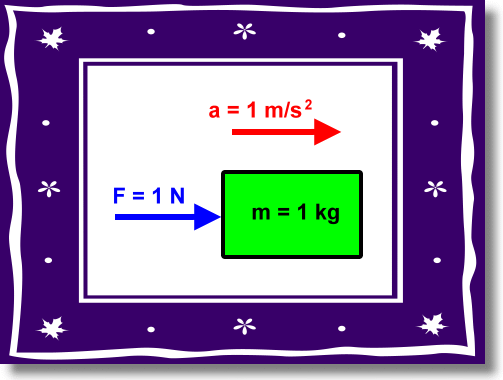# Define one newton force

Define one newton force

One Newton is 1 kilogram meter per second square. It is the SI unit of force. It is the force needed to accelerate a mass of 1 kilogram by 1 m/s2 in the direction of the applied force.